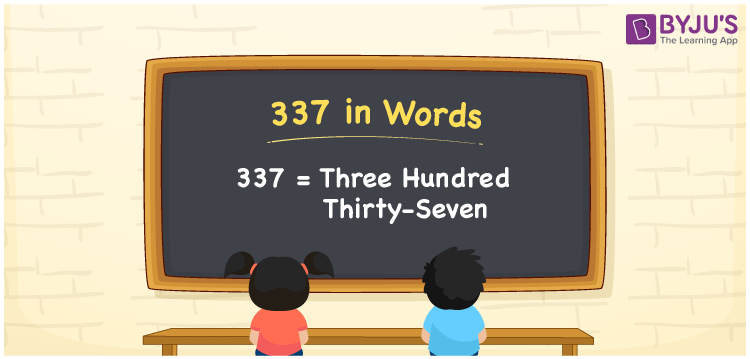# 337 in Words

The number 337 in words is “three hundred thirty-seven”. The numeral 337 has three-digits and its place values help to convert the number 337 into words. Also, the number 337 is a cardinal number. For example, the cost of 2 kg apples is Rs. 337.

 337 in Words: Three Hundred Thirty-seven. Three Hundred Thirty-seven in Numerical Form: 337.

## 337 in English Words## How to Write 337 in Words?

The below table depicts the place values of the natural number 337.

 Hundreds Tens Ones 3 3 7

The expanded form of 337 is as follows:

= 3 × Hundred + 3 × Ten + 7 × One

= 3 × 100 + 3 × 10 + 7 × 1

= 300 + 30 + 7

= 337

= Three hundred thirty-seven

Hence, 337 in words is three hundred thirty-seven.

337 in words – Three hundred thirty-seven

Is 337 an odd number? – Yes

Is 337 an even number? – No

Is 337 a perfect square number? – No

Is 337 a perfect cube number? – No

Is 337 a prime number? – Yes

Is 337 a composite number? – No

## Frequently Asked Questions on 337 in Words

Q1

### How to write 337 in words?

337 in words is three hundred thirty-seven.

Q2

### Simplify 300 + 37, and express it in words.

Simplifying 300 + 37, we get 337. Hence, 337 in words is three hundred thirty-seven.

Q3

### Is 337 a prime number?

Yes, 337 is a prime number.